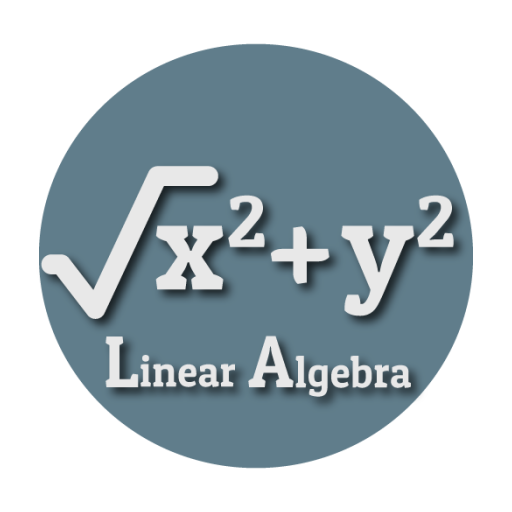#### Current Version : 1.1 Downloads : 16,611 Size : 8.2 MB

Linear Algebra is an app to solve some of the mathematical problems on the go. Here in this app, you need to enter the value and click the Calculate button to get the result. You can find some of the fundamental theorems of Linear Algebra and its calculation can be done in the app. The app is very easy to use and free to download. It has a very simple and easy to use UI. All the formulas given here are easy to understand and a quick introduction to linear algebra methods is given. You can generate random values for the matrices and calculate the result.

Some of the key calculations available in the app are:
<b>Matrices</b>
- Subtraction of Matrix
- Matrix Multiplication
- Transpose of Matrix
- Trace of Matrix
- Determinant of Matrix

<b>Linear Equation</b>
- Gaussian Jordan Method
- Gaussian Elimination

<b>Vector Space</b>
- Subtraction of Vector
- Scalar Product
- Projection
- Vectorial Product
- An angle between the Vector
- Orthogonal Vectors

<b>Geometry</b>
- Cone
- Cylinder
- Isosceles Triangle
- Equilateral Triangle
- Square
- Sphere
- Rectangle
- Rhombus
- Parallelogram
- Trapezoid

--------------------------------------------------------------------------------------------------
This App is developed at ASWDC by Janvi Raiyani (160540107118) and Monali Gujarati (140540107045), CE Student. ASWDC is Apps, Software, and Website Development Center @ Darshan Institute of Engineering & Technology, Rajkot run by Students & Staff of Computer Engineering Department

Call us: +91-9727747317

Write to us: aswdc@darshan.ac.in
Visit: http://www.darshan.ac.in http://www.darshan.ac.in/aswdc

ASWDC is established by Department of Computer Engineering where students work on live projects under guidance of staff and industry experts. Students are getting extensive knowledge and industrial experience of cutting edge technologies. ASWDC fills gap between academic curriculum and industry expectation.